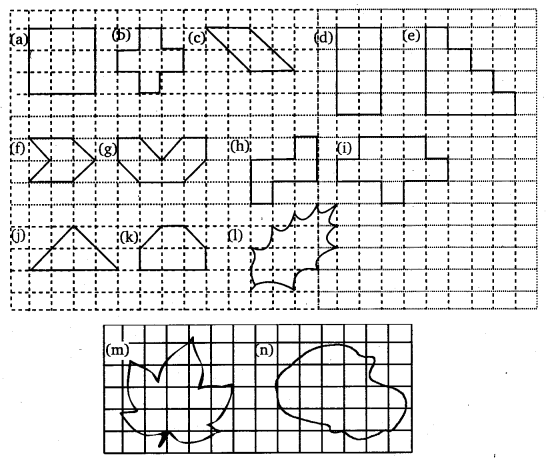# Class 6 Maths NCERT Solutions for Chapter 10 Mensuration Ex – 10.2

## Mensuration

Question 1.
Find the areas of the following figures by counting square:Solution:
Placing these figures on the centimetre square, we have
(a) Full squares = 9
∴ Area covered by the figure = 9 x 1 sq. cm = 9 sq. cm

(b) Full squares = 5
∴ Area covered by the figure = 5 x 1 sq. cm = 5 sq. cm

(c) Full squares = 2
Half squares = 4
∴ Area covered by the figure$\left( 2\times 1+4\times \frac { 1 }{ 2 } \right)$ sq. cm
= (2 + 2) sq cm = 4 sq. cm

(d) Full squares = 10
∴ Area covered by the figure = 10 x 1 sq. cm = 10 sq. cm

(e) Full squares = 10
∴ Area covered by the figure = 10 x 1 sq. cm = 10 sq. cm

(f) Full squares = 2
Half squares = 4
∴ Area covered by the figure =$\left( 2\times 1+4\times \frac { 1 }{ 2 } \right)$ sq. cm
= (2 + 2)sq. cm = 4 sq. cm

(g) Full squares = 4
Half squares = 4
∴ Area covered by the figure =$\left( 2\times 1+4\times \frac { 1 }{ 2 } \right)$ sq. cm
= (4 + 2)sq. cm = 6 sq. cm

(h) Full squares = 5
∴ Area covered by the figure = 5 x 1 sq. cm
= 5 sq. cm

(i) Full squares = 9
∴ Area covered by the figure = 9 x 1 sq. cm
= 9 sq. cm

(j) Full squares = 2
Half squares = 4
∴ Area covered by the figure =$\left( 2\times 1+4\times \frac { 1 }{ 2 } \right)$ sq. cm
= (2 + 2)sq. cm = 4 sq. cm

(k) Full squares = 4
Half squares = 2
∴ Area covered by the figure =$\left( 4\times 1+2\times \frac { 1 }{ 2 } \right)$ sq. cm
= (4 + 1)sq. cm = 5 sq. cm

(l) Full squares = 4
More than half squares = 3
Half square = 2
∴ Area covered by the figure =$\left( 4\times 1+3\times 1+2\times \frac { 1 }{ 2 } \right)$ sq. cm
= (4 + 3 + 1)sq. cm
= 8 sq. cm

(m) Full squares = 7
More than half squares = 7
Half square = 0
∴ Area covered by the figure =$\left( 7\times 1+7\times 1+0\times \frac { 1 }{ 2 } \right)$ sq. cm
= (7 + 7 + 0)sq. cm
= 14 sq. cm

(n) Full squares = 10
More than half squares = 8
Half square = 0
∴ Area covered by the figure =$\left( 10\times 1+8\times 1+0\times \frac { 1 }{ 2 } \right)$ sq. cm
= (10 + 8 + 0)sq. cm
= 18 sq. cm